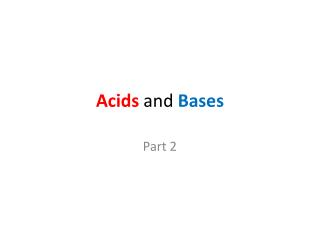DownloadDownload PresentationAcids and Bases

# Acids and Bases

Download Presentation## Acids and Bases

- - - - - - - - - - - - - - - - - - - - - - - - - - - E N D - - - - - - - - - - - - - - - - - - - - - - - - - - -
##### Presentation Transcript

1. Acids and Bases Part 2

2. Conceptual Definitions Arrhenius’s Concept - acids are substances that produce hydrogen ions, when dissolvedin water. HCl(g) + H2O -------> H3O 1+(aq) + Cl1-(aq) Bases are substances that produce hydroxide ions when dissolved in water. NaOH(s) + H2O------> Na1+(aq)+ OH1-(aq)

3. Limitations to Arrhenius Theory • According to Arrhenius, ionic compounds dissolved in water should have a neutral pH.This isn’t always true. • Consider the following salts: • Na2CO3 = gives a basic pH in water • NaH2PO4 = gives an acidic pH in water

4. Limitations to Arrhenius Theory • According to Arrhenius, onlyH1+-, and OH1- -containing compounds can be acids orbases.This isn’t always true. • Consider the following: • NH3(g) + H2O(l) NH4+(aq) + OH-(aq) • Here ammonia (which does not contain an OH1- ) reactswith water to produce the characteristic hydroxide.

5. Bronsted’s Definition of Acids and Bases: Acidsdonateprotons (hydrogen ions); Basesacceptprotons (hydrogen ions). -explains the interaction of H2O & ions The following is the example of HCl dissolved in water, to give the following ionization equation: HCl(aq) + H2O(l)H3O+(aq) + Cl-(aq) HCl(aq) is an acid because it donates protons to H2O molecules H2O is a base because it accepts protons from HCl molecules.

6. Bronsted’s Definition of Acids & Bases: -explains the basicity of NH3, NH3(g) + H2O(l) NH4+(aq) + OH-(aq) H2O donates a proton (hydrogen ion) and is an acid. The lone electron pair on the NH3 accepts a proton (hydrogen-ion) from water and is a base. The resulting solution will contain a free hydroxide ion.

7. Strength of Acids and Bases is determined by the degree to which a substance produces ions in solution. • A strong acid or base is a substance which completely ionizes. • In other words if 100 molecules of a strong acid like HCl are placed in water all 100 of them will react with H2O producing 100 H3O1+ ions and 100 Cl1- ions. • Weak acids and bases only partially ionize.

8. Strong Acid - the reaction below goes to completion. HCl(g) + H2O --------> H3O+(aq) + Cl1-(aq) Weak Acid - the reaction occurs to a limited extent. In the example below if 100 acetic acid molecules are placed in water only a few of them will successfully react with water molecules producing hydroniumions (H3O+). Most CH3COOH molecules remain intact. CH3COOH + H2O H3O+(aq)+ CH3COO1-(aq)

9. Strong Acids in order of decreasing strength are HClO4, HI, HBr, H2SO4, HCl, HNO3 Acid strength has to do with the ease with which an acid can lose a proton. If the binary acid strengths (HI, HBr, HCl) are compared it can be seen that HI is the strongest acid of this group because its iodide ion is the largest of the group so the force between the hydrogen ion and the iodide ion is the weakest so it loses its proton most easily.

10. Force is strongest since the ions are closest Br1- H1+ H1+ H1+ Cl1- Force is weakest since the ions are furthest Remember the weaker the force the stronger the acid I1-

11. Strong Basesinclude hydroxides of group 1A metals and Ca2+, Ba2+, and Sr2+. As with acids, the weaker the bonds, the stronger the base since liberation of OH1- ions is easiest when the bonds are weakest.

12. Polyprotic Acids donate protons in steps. For instance carbonic acid, H2CO3 has two protons to donate and it does this in two steps: step 1: H2CO3 + H2O HCO31- + H3O+ step 2: HCO31- + H2O CO32- + H3O+ note: The arrows are constructed in this manner to show the reverse reaction has a greater tendency than the forward reaction.

13. conjugate pair conjugate pair Conjugate Acid - Base Pairs –These are pairs of molecules that differ by 1 hydrogen atom in an acid-base reversible reaction. For instance when sulfurous acid, H2SO3 reacts with water the following equilibrium is established: acid base acid base H2SO3 + H2O H3O++ HSO31-

14. conjugate pair conjugate pair acid baseacidbase H2SO3 + H2OH3O++ HSO31- In the forward direction the H2SO3 is the proton donor so it’s the acid and the H2O is the proton acceptorso it’s thebase. In the reverse direction the H3O+is the proton donor so it’s the acid and the HSO31- is the proton acceptorso it’s a base.

15. conjugate pair conjugate pair acid base acid base H2SO3 + H2O H3O++ HSO31- When looking at both forward and reverse reactions it is easy to pick out a pair of molecules which differ by a single proton (H atom without its electron). These pairs are called conjugate acid-base pairs.

16. Identify the acid and conjugate base and the base and conjugate acid in the two equations below: HCl(aq) + H2O(l)H3O+(aq) + Cl-(aq) NH3(g) + H2O(l) NH4+(aq) + OH-(aq) NOTE: water behaves as either an acid or a base depending on the context, we say it is AMPHIPROTIC Acid Base C.A. C.B. Base Acid C.A. C.B.

17. STUFF YOU SHOULD KNOW • Arrhenius vsBronsted theory • Difference between strong acid and weak acid • How to determine conjugate acid and conjugate bases • Know what ionization is